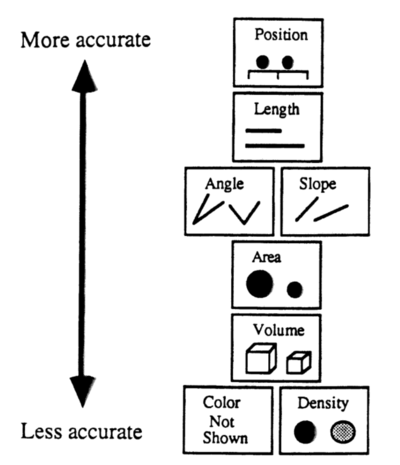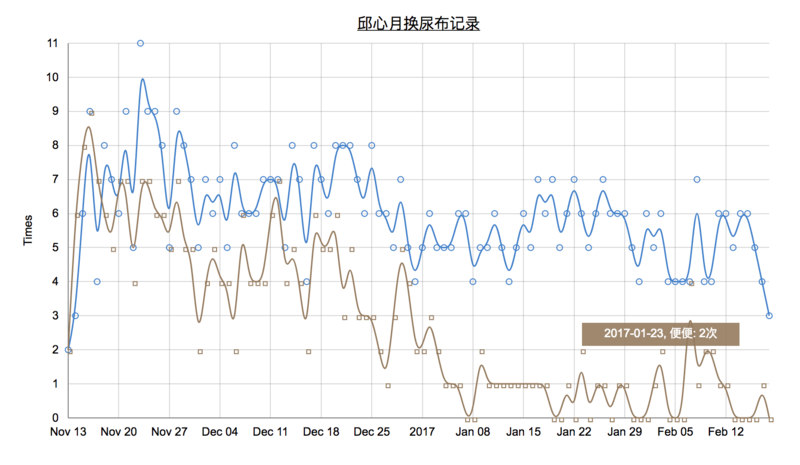## 数据来源¶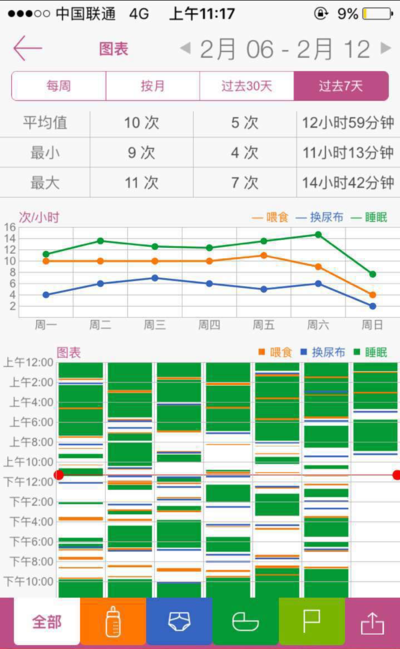### 数据准备¶

`Baby Tracker`导出的数据是一些CSV文件组成是压缩包，解压之后大致结果是这样的：

• 哺乳记录
• 睡眠记录
• 换尿布记录
• 喂药/体温记录
• 里程碑记录

#### python + pandas¶

``````name,date,status,note

``````

``````date,urinate,stool
2016-11-13,2,2
2016-11-14,3,6
2016-11-15,6,8
``````

``````import numpy as np
import pandas as pd

diaper['date'] = pd.to_datetime(diaper['date'], format='%Y/%m/%d %H:%M')
diaper.index=diaper['date']

def mapper(key):
if key == '嘘嘘':
return pd.Series([1, 0], index=['urinate', 'stool'])
elif key == '便便':
return pd.Series([0, 1], index=['urinate', 'stool'])
else:
return pd.Series([1, 1], index=['urinate', 'stool'])

converted = diaper['status'].apply(mapper)
diaper = pd.concat([diaper, converted], axis=1)

grouped = diaper.groupby(pd.TimeGrouper('D'))

result = grouped.aggregate(np.sum)
result.to_csv('data/diaper_normolized.csv')
``````

### 可视化¶

#### 尝试1 - 曲线图¶

``````var valueline = d3.svg.line()
.x(function(d) {
return x(d.date);
})
.y(function(d) {
return y(d.urinate);
});

var stoolline = d3.svg.line()
.x(function(d) {
return x(d.date);
})
.y(function(d) {
return y(d.stool);
});
``````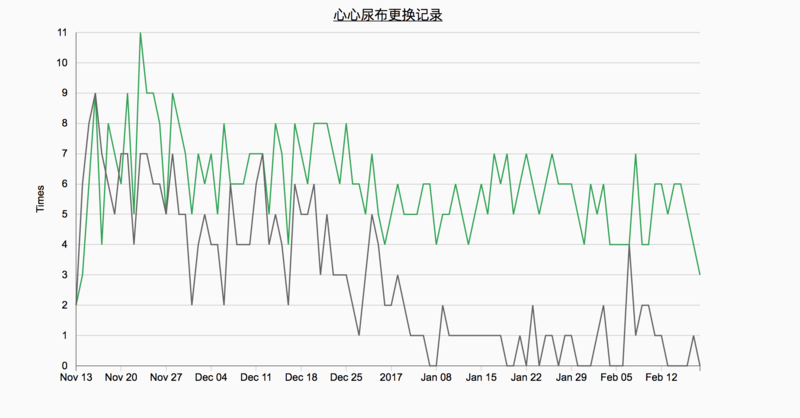``````var valueline = d3.svg.line()
.interpolate('basis')
.x(function(d) {
return x(d.date);
})
.y(function(d) {
return y(d.urinate);
});
``````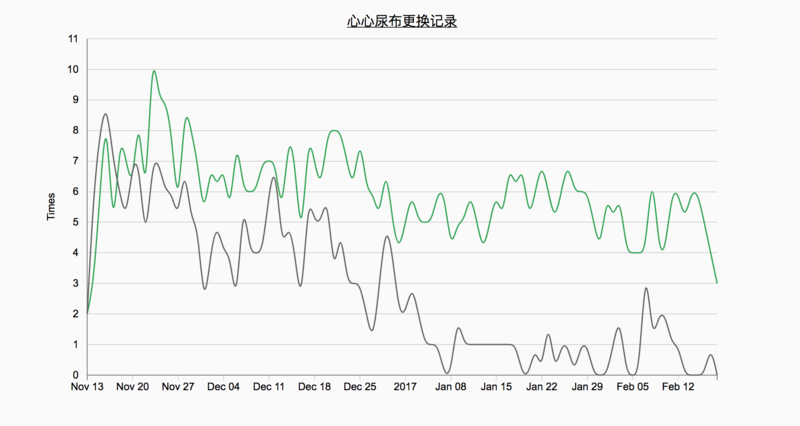#### 尝试2 - 散点图（气泡图）¶

``````svg.selectAll("dot")
.data(data)
.enter().append("circle")
.attr('stroke', '#FD8D3C')
.attr('fill', '#FD8D3C')
.attr('opacity', ".7")
.attr("r", function(d) {return 3;})
.attr("cx", function(d) { return x(d.date); })
.attr("cy", function(d) { return y(d.urinate); });
``````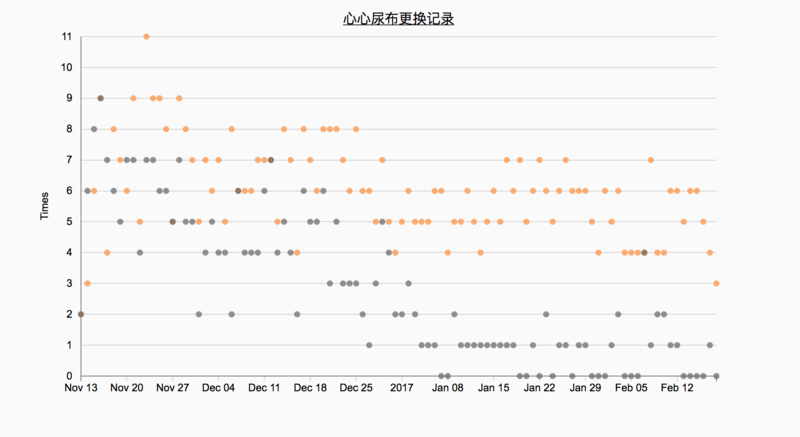``````svg.selectAll("dot")
.data(data)
.enter().append("circle")
.attr('stroke', '#FD8D3C')
.attr('fill', '#FD8D3C')
.attr('opacity', function(d) {return d.urinate * 0.099})
.attr("r", function(d) {return d.urinate;})
.attr("cx", function(d) { return x(d.date); })
.attr("cy", function(d) { return y(d.urinate); })
.on("mouseover", function(d) {
div.html(formatTime(d.date) + ", 嘘嘘: "  + d.urinate + "次")
.style("left", (d3.event.pageX) + "px")
.style("top", (d3.event.pageY - 28) + "px")
.style("opacity", .9)
.style("background", "#FD8D3C");
})
.on("mouseout", function(d) {
div.style("opacity", 0);
});
``````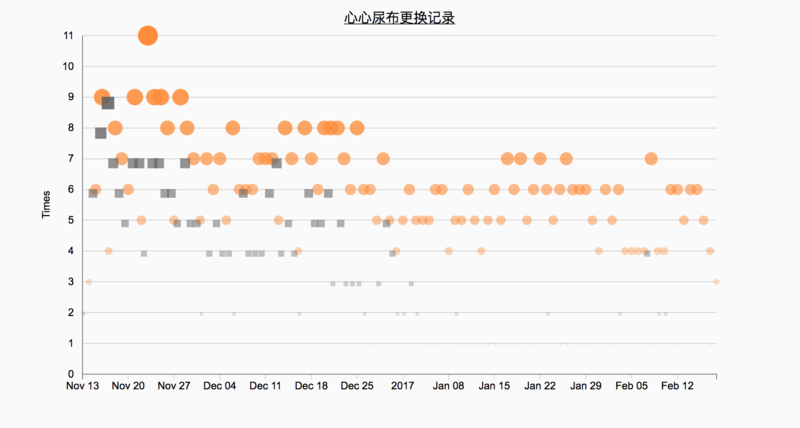### 一点理论¶

1. 明确可视化的目的
2. 数据获取
3. 数据预处理
4. 选择合适的图表
5. 结果呈现

#### 视觉编码（Visual Encoding）¶

1. 位置
2. 形状（体积）
3. 纹理
4. 角度
5. 长度
6. 色彩
7. 色彩饱和度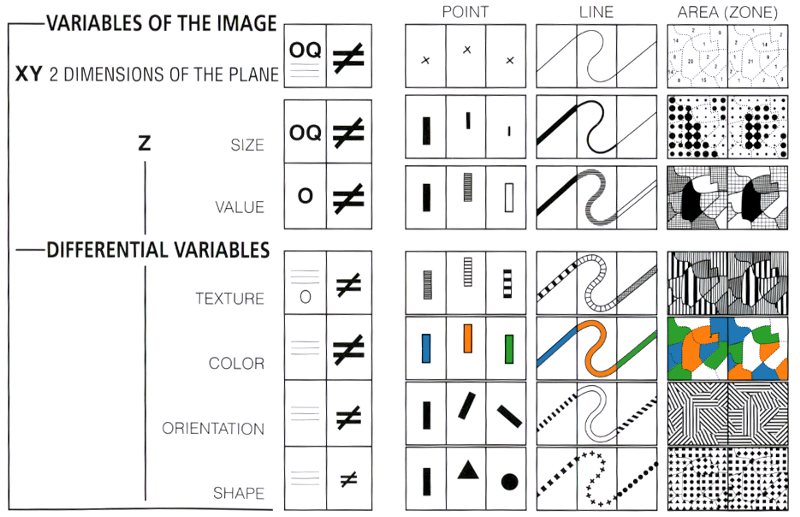Within the plane a mark can be at the top or the bottom, to the right or the left. The eye perceives two independent dimensions along X and Y, which are distinguished orthogonally. A variation in light energy produces a third dimension in Z, which is independent of X and Y…

The eye is sensitive, along the Z dimension, to 6 independent visual variables, which can be superimposed on the planar figures: the size of the marks, their value, texture, color, orientation, and shape. They can represent differences (≠), similarities (≡), a quantified order (Q), or a nonquantified order (O), and can express groups, hierarchies, or vertical movements.

Jock Mackinlay发表过关于不同视觉编码优先级的研究：